# NCERT Solutions For Class 6 Maths Practical Geometry Exercise 14.5

NCERT Solutions For Class 6 Maths Practical Geometry Exercise 14.5

### NCERT Solutions For Class 6 Maths Practical Geometry Exercise 14.5

NCERT Solutions For Class 6 Maths Chapter 14 Practical Geometry Ex 14.5

Exercise 14.5

Ex 14.5 Class 6 Maths Question 1.
Draw AB of length 7.3 cm and find its axis of symmetry.
Solution: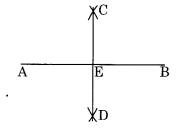Ex 14.5 Class 6 Maths Question 2.
Draw a line segment of length 9.5 cm and construct its perpendicular bisector.
Solution: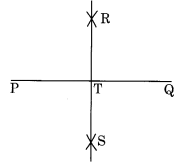Step II: With centres P and Q and radius more than half of PQ, draw two arcs which meet each other at R and S.Thus, RS is the perpendicular bisector of PQ.

Ex 14.5 Class 6 Maths Question 3.Step II : With centre X and Y and radius more than half of XY, draw two arcs which meet each other at U and V.Ex 14.5 Class 6 Maths Question 4.
Draw a line segment of length 12.8 cm. Using compasses, divide it into four equal parts. Verify by actual measurement.
Solution: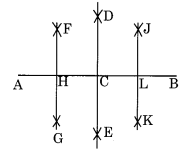Step II : With centre A and B and radius more than half of AB, draw two arcs which meet each other at D and E.Ex 14.5 Class 6 Maths Question 5.Ex 14.5 Class 6 Maths Question 6.Ex 14.5 Class 6 Maths Question 7.Solution:
Step I: Draw a circle with centre C and radius 3.4 cm.
Step II : Draw a diameter AB of the circle.Ex 14.5 Class 6 Maths Question 8.
Draw a circle of radius 4 cm. Draw any two of its chords. Construct the perpendicular bisectors of these chords. Where do they meet?
Solution:
Step I: Draw a circle with centre 0 and radius 4 cm.Ex 14.5 Class 6 Maths Question 9.Solution:
Step I: Draw an angle XOY with O as its vertex.
Step II : Take any point A on OY and B on OX, such that OA + OB.Step III : Draw the perpendicular bisectors of OA and OB which meet each other at a point P.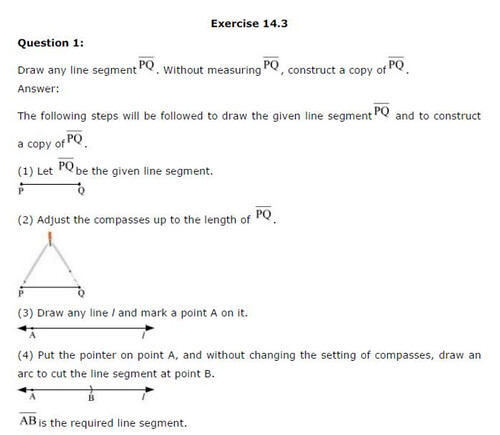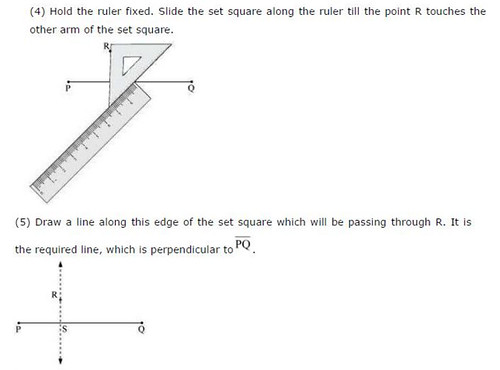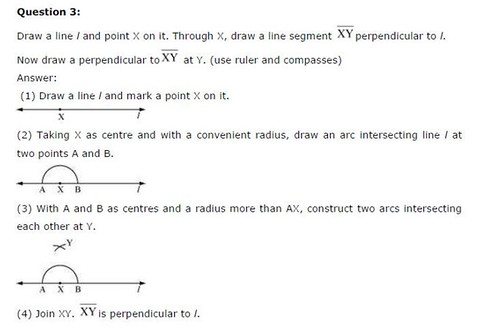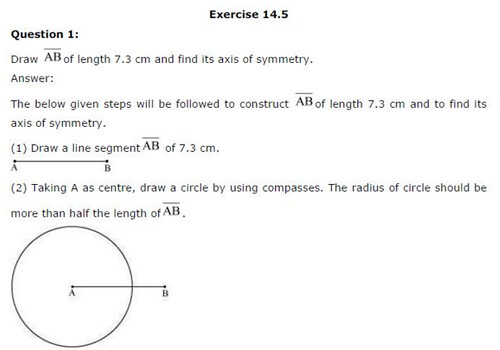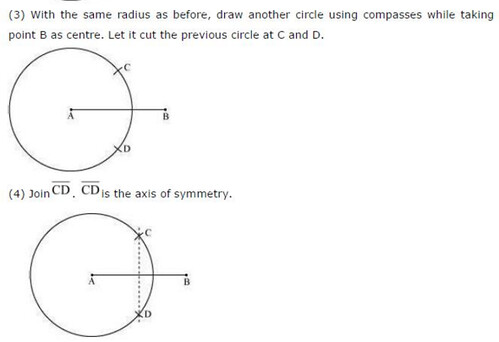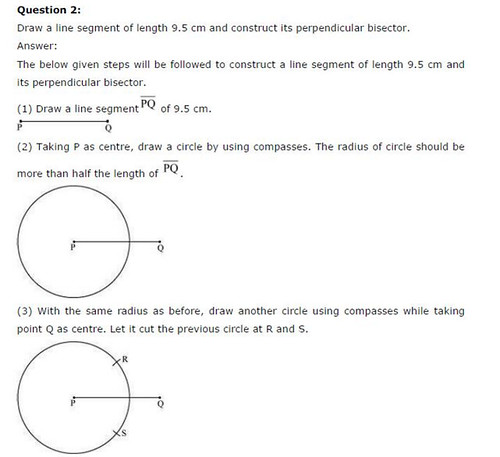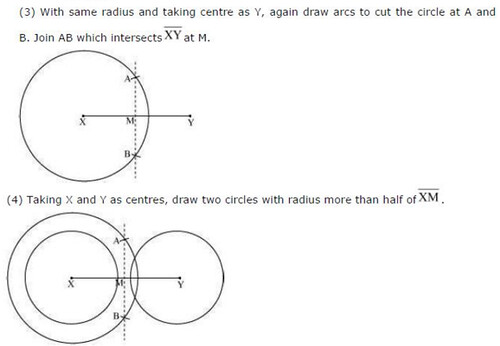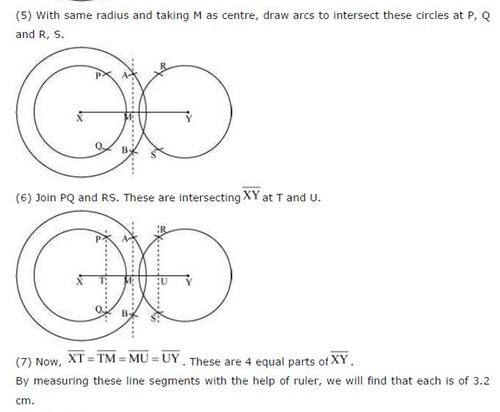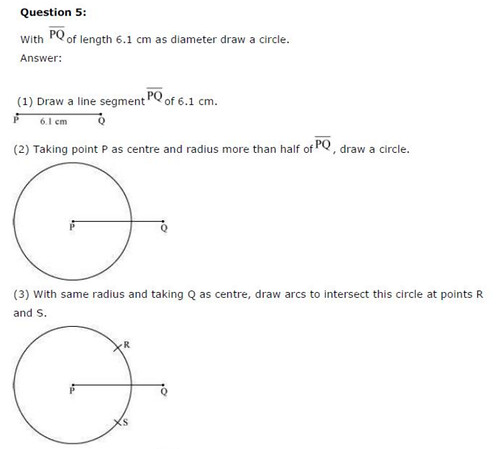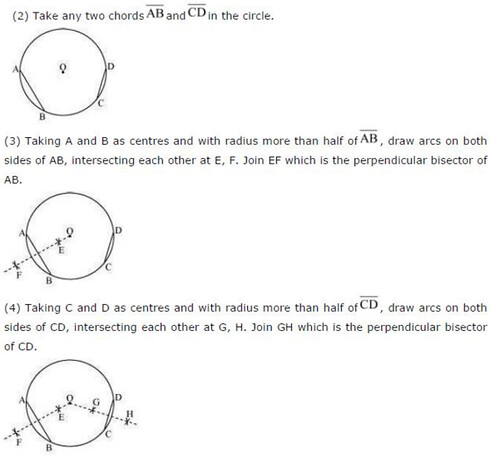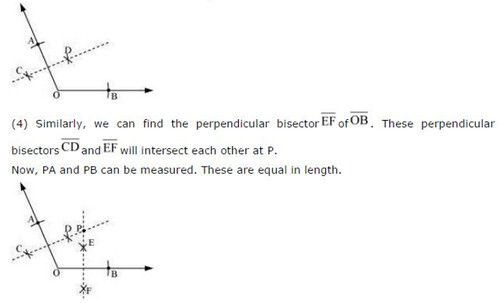## SabDekho

The Complete Educational Website